##### SAT: 1,001 Practice Questions For DummiesYou'll probably encounter several questions on the SAT Math exam that require you to work with exponents. The following practice questions deal with like bases, fractions, and exponents in parentheses!

## Practice questions

1. In this equation, what is the value of xy?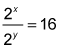A. 2 B. 3 C. 4 D. 5
2. What is the value of a in the equation if b2 = 5 and a < 0?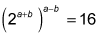A. –1 B. –2 C. –3 D. –4

1. The correct answer is Choice (C). Start with the fraction and subtract the exponents, just as you'd do to divide any other terms with like bases: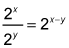You know that 16 equals 24, so set 24 equal to the 2 with the subtracted exponents: 2xy = 24 Therefore xy = 4.
2. The correct answer is Choice (C). Simplify the exponential expression by multiplying the exponents, just as you'd do for any other base with an exponent of an exponent: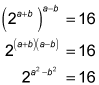You know that 16 equals 24, so set 24 equal to the 2 with the subtracted exponents: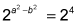Therefore, a2b2 = 4. You know that b2 = 5, so plug in the value of b2: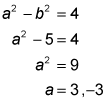You also know that a < 0, so a has to be –3.